Skip to main content
$$\newcommand{\id}{\mathrm{id}}$$ $$\newcommand{\Span}{\mathrm{span}}$$ $$\newcommand{\kernel}{\mathrm{null}\,}$$ $$\newcommand{\range}{\mathrm{range}\,}$$ $$\newcommand{\RealPart}{\mathrm{Re}}$$ $$\newcommand{\ImaginaryPart}{\mathrm{Im}}$$ $$\newcommand{\Argument}{\mathrm{Arg}}$$ $$\newcommand{\norm}{\| #1 \|}$$ $$\newcommand{\inner}{\langle #1, #2 \rangle}$$ $$\newcommand{\Span}{\mathrm{span}}$$

# 1.2: Trigonometric Functions of an Acute Angle

•• Contributed by Michael Corral
• Professor (Mathematics) at Schoolcraft College

$$\newcommand{\vecs}{\overset { \rightharpoonup} {\mathbf{#1}} }$$

$$\newcommand{\vecd}{\overset{-\!-\!\rightharpoonup}{\vphantom{a}\smash {#1}}}$$

Consider a right triangle $$\triangle\,ABC$$, with the right angle at $$C$$ and with lengths $$a$$, $$b$$, and $$c$$, as in the figure on the right. For the acute angle $$A$$, call the leg $$\overline{BC}$$ its opposite side, and call the leg $$\overline{AC}$$ its adjacent side. Recall that the hypotenuse of the triangle is the side $$\overline{AB}$$. The ratios of sides of a right triangle occur often enough in practical applications to warrant their own names, so we define the six trigonometric functions of $$A$$ as follows: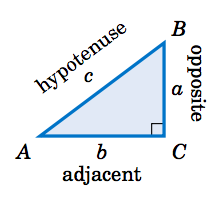Table 1.2 The six trigonometric functions of $$A$$We will usually use the abbreviated names of the functions. Notice from Table 1.2 that the pairs $$\sin A$$ and $$\csc A$$, $$\cos A$$ and $$\sec A$$, and $$\tan A$$ and $$\cot A$$ are reciprocals: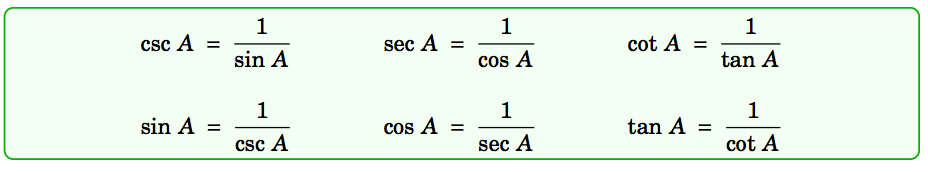Example 1.5

For the right triangle $$\triangle\,ABC$$ shown on the right, find the values of all six trigonometric functions of the acute angles $$A$$ and $$B$$.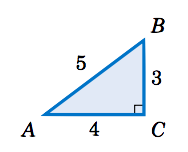Solution:

The hypotenuse of $$\triangle\,ABC$$ has length $$5$$. For angle $$A$$, the opposite side $$\overline{BC}$$ has length $$3$$ and the adjacent side $$\overline{AC}$$ has length $$4$$. Thus:

$\nonumber \sin A ~=~ \dfrac{\text{opposite}}{\text{hypotenuse}} ~=~ \dfrac{3}{5} \qquad\qquad \cos A ~=~ \dfrac{\text{adjacent}}{\text{hypotenuse}} ~=~ \dfrac{4}{5} \qquad\qquad \tan A ~=~ \dfrac{\text{opposite}}{\text{adjacent}} ~=~ \dfrac{3}{4}$

$\nonumber \csc A ~=~ \dfrac{\text{hypotenuse}}{\text{opposite}} ~=~ \dfrac{5}{3} \qquad\qquad \sec A ~=~ \dfrac{\text{hypotenuse}}{\text{adjacent}} ~=~ \dfrac{5}{4} \qquad\qquad \cot A ~=~ \dfrac{\text{adjacent}}{\text{opposite}} ~=~ \dfrac{4}{3}$

For angle $$B$$, the opposite side $$\overline{AC}$$ has length $$4$$ and the adjacent side $$\overline{BC}$$ has length $$3$$. Thus:

$\sin B ~=~ \dfrac{\text{opposite}}{\text{hypotenuse}} ~=~ \dfrac{4}{5} \qquad\qquad \cos B ~=~ \dfrac{\text{adjacent}}{\text{hypotenuse}} ~=~ \dfrac{3}{5} \qquad\qquad \tan B ~=~ \dfrac{\text{opposite}}{\text{adjacent}} ~=~ \dfrac{4}{3}$

$\csc B ~=~ \dfrac{\text{hypotenuse}}{\text{opposite}} ~=~ \dfrac{5}{4} \qquad\qquad \sec B ~=~ \dfrac{\text{hypotenuse}}{\text{adjacent}} ~=~ \dfrac{5}{3} \qquad\qquad \cot B ~=~ \dfrac{\text{adjacent}}{\text{opposite}} ~=~ \dfrac{3}{4}$

Notice in Example 1.5 that we did not specify the units for the lengths. This raises the possibility that our answers depended on a triangle of a specific physical size.

For example, suppose that two different students are reading this textbook: one in the United States and one in Germany. The American student thinks that the lengths $$3$$, $$4$$, and $$5$$ in Example 1.5 are measured in inches, while the German student thinks that they are measured in centimeters. Since $$1$$ in $$\approx$$ $$2.54$$ cm, the students are using triangles of different physical sizes (see Figure 1.2.1 below, not drawn to scale).Figure 1.2.1: $$△ ABC ∼ △ A ′B ′C ′$$

If the American triangle is $$\triangle\,ABC$$ and the German triangle is $$\triangle\,A'B'C'$$, then we see from Figure 1.2.1 that $$\triangle\,ABC$$ is similar to $$\triangle\,A'B'C'$$, and hence the corresponding angles are equal and the ratios of the corresponding sides are equal. In fact, we know that common ratio: the sides of $$\triangle\,ABC$$ are approximately $$2.54$$ times longer than the corresponding sides of $$\triangle\,A'B'C'$$. So when the American student calculates $$\sin A$$ and the German student calculates $$\sin A'$$, they get the same answer:

$\triangle\,ABC ~\sim~ \triangle\,A'B'C' \quad\Rightarrow\quad \dfrac{BC}{B'C'} ~=~ \dfrac{AB}{A'B'} \quad\Rightarrow\quad \dfrac{BC}{AB} ~=~ \dfrac{B'C'}{A'B'} \quad\Rightarrow\quad \sin A ~=~ \sin A'$

Likewise, the other values of the trigonometric functions of $$A$$ and $$A'$$ are the same. In fact, our argument was general enough to work with any similar right triangles. This leads us to the following conclusion:

When calculating the trigonometric functions of an acute angle $$A$$, you may use any right triangle which has $$A$$ as one of the angles.

Since we defined the trigonometric functions in terms of ratios of sides, you can think of the units of measurement for those sides as canceling out in those ratios. This means that the values of the trigonometric functions are unitless numbers. So when the American student calculated $$3/5$$ as the value of $$\sin A$$ in Example 1.5, that is the same as the $$3/5$$ that the German student calculated, despite the different units for the lengths of the sides.

Example 1.6

Find the values of all six trigonometric functions of $$45^\circ$$.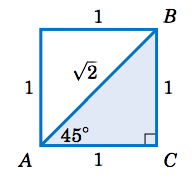Solution:

Since we may use any right triangle which has $$45^\circ$$ as one of the angles, use the simplest one: take a square whose sides are all $$1$$ unit long and divide it in half diagonally, as in the figure on the right. Since the two legs of the triangle $$\triangle\,ABC$$ have the same length, $$\triangle\,ABC$$ is an isosceles triangle, which means that the angles $$A$$ and $$B$$ are equal. So since $$A + B = 90^\circ$$, this means that we must have $$A = B = 45^\circ$$. By the Pythagorean Theorem, the length $$c$$ of the hypotenuse is given by

$c^2 ~=~ 1^2 ~+~ 1^2 ~=~ 2 \quad\Rightarrow\quad c ~=~ \sqrt{2} ~$

Thus, using the angle $$A$$ we get:

$\sin\;45^\circ ~=~ \dfrac{\text{opposite}}{\text{hypotenuse}} ~=~ \dfrac{1}{\sqrt{2}} \quad\quad \cos\;45^\circ ~=~ \dfrac{\text{adjacent}}{\text{hypotenuse}} ~=~ \dfrac{1}{\sqrt{2}} \quad\quad \tan\;45^\circ ~=~ \dfrac{\text{opposite}}{\text{adjacent}} ~=~ \dfrac{1}{1} ~=~ 1$

$\csc\;45^\circ ~=~ \dfrac{\text{hypotenuse}}{\text{opposite}} ~=~ \sqrt{2} \quad\quad \sec\;45^\circ ~=~ \dfrac{\text{hypotenuse}}{\text{adjacent}} ~=~ \sqrt{2} \quad\quad \cot\;45^\circ ~=~ \dfrac{\text{adjacent}}{\text{opposite}} ~=~ \dfrac{1}{1} ~=~ 1$

Note that we would have obtained the same answers if we had used any right triangle similar to $$\triangle\,ABC$$. For example, if we multiply each side of $$\triangle\,ABC$$ by $$\sqrt{2}$$, then we would have a similar triangle with legs of length $$\sqrt{2}$$ and hypotenuse of length $$2$$. This would give us $$\sin 45^\circ = \frac{\sqrt{2}}{2}$$, which equals $$\frac{\sqrt{2}}{\sqrt{2} \cdot \sqrt{2}} = \frac{1}{\sqrt{2}}$$ as before. The same goes for the other functions.

Example 1.7

Find the values of all six trigonometric functions of $$60^\circ$$.Solution:

Since we may use any right triangle which has $$60^\circ$$ as one of the angles, we will use a simple one: take a triangle whose sides are all $$2$$ units long and divide it in half by drawing the bisector from one vertex to the opposite side, as in the figure on the right. Since the original triangle was an equilateral triangle (i.e. all three sides had the same length), its three angles were all the same, namely $$60^\circ$$. Recall from elementary geometry that the bisector from the vertex angle of an equilateral triangle to its opposite side bisects both the vertex angle and the opposite side. So as in the figure on the right, the triangle $$\triangle\,ABC$$ has angle $$A = 60^\circ$$ and angle $$B = 30^\circ$$, which forces the angle $$C$$ to be $$90^\circ$$. Thus, $$\triangle\,ABC$$ is a right triangle. We see that the hypotenuse has length $$c = AB = 2$$ and the leg $$\overline{AC}$$ has length $$b = AC = 1$$. By the Pythagorean Theorem, the length $$a$$ of the leg $$\overline{BC}$$ is given by

$a^2 ~+~ b^2 ~=~ c^2 \quad\Rightarrow\quad a^2 ~=~ 2^2 ~-~ 1^2 ~=~ 3 \quad\Rightarrow\quad a ~=~ \sqrt{3} ~.$

Thus, using the angle $$A$$ we get:

$\sin 60^\circ \;=\; \dfrac{\text{opposite}}{\text{hypotenuse}} \;=\; \dfrac{\sqrt{3}}{2} \qquad \cos 60^\circ \;=\; \dfrac{\text{adjacent}}{\text{hypotenuse}} \;=\; \dfrac{1}{2} \qquad \tan 60^\circ \;=\; \dfrac{\text{opposite}}{\text{adjacent}} \;=\; \dfrac{\sqrt{3}}{1} \,=\, \sqrt{3}$

$\csc 60^\circ \;=\; \dfrac{\text{hypotenuse}}{\text{opposite}} \;=\; \dfrac{2}{\sqrt{3}} \qquad \sec 60^\circ \;=\; \dfrac{\text{hypotenuse}}{\text{adjacent}} \;=\; 2 \qquad \cot 60^\circ \;=\; \dfrac{\text{adjacent}}{\text{opposite}} \;=\; \dfrac{1}{\sqrt{3}}~\quad\quad$

Notice that, as a bonus, we get the values of all six trigonometric functions of $$30^\circ$$, by using angle $$B = 30^\circ$$ in the same triangle $$\triangle\,ABC$$ above:

$\sin 30^\circ \;=\; \dfrac{\text{opposite}}{\text{hypotenuse}} \;=\; \dfrac{1}{2} \qquad \cos 30^\circ \;=\; \dfrac{\text{adjacent}}{\text{hypotenuse}} \;=\; \dfrac{\sqrt{3}}{2} \qquad \tan 30^\circ \;=\; \dfrac{\text{opposite}}{\text{adjacent}} \;=\; \dfrac{1}{\sqrt{3}}\quad\quad$

$\csc 30^\circ \;=\; \dfrac{\text{hypotenuse}}{\text{opposite}} \;=\; 2 \qquad \sec 30^\circ \;=\; \dfrac{\text{hypotenuse}}{\text{adjacent}} \;=\; \dfrac{2}{\sqrt{3}} \qquad \cot 30^\circ \;=\; \dfrac{\text{adjacent}}{\text{opposite}} \;=\; \dfrac{\sqrt{3}}{1} \;=\; \sqrt{3}$

Example 1.8

$$A$$ is an acute angle such that $$\sin A = \frac{2}{3}$$. Find the values of the other trigonometric functions of $$A$$.Solution:

In general it helps to draw a right triangle to solve problems of this type. The reason is that the trigonometric functions were defined in terms of ratios of sides of a right triangle, and you are given one such function (the sine, in this case) already in terms of a ratio: $$\sin\;A = \frac{2}{3}$$. Since $$\sin\;A$$ is defined as $$\frac{\text{opposite}}{\text{hypotenuse}}$$, use $$2$$ as the length of the side opposite $$A$$ and use $$3$$ as the length of the hypotenuse in a right triangle $$\triangle\,ABC$$ (see the figure above), so that $$\sin\;A = \frac{2}{3}$$. The adjacent side to $$A$$ has unknown length $$b$$, but we can use the Pythagorean Theorem to find it:

$2^2 ~+~ b^2 ~=~ 3^2 \quad\Rightarrow\quad b^2 ~=~ 9 ~-~ 4 ~=~ 5 \quad\Rightarrow\quad b ~=~ \sqrt{5}$

We now know the lengths of all sides of the triangle $$\triangle\,ABC$$, so we have:

$\cos\;A \;=\; \dfrac{\text{adjacent}}{\text{hypotenuse}} \;=\; \dfrac{\sqrt{5}}{3} \qquad \tan\;A \;=\; \dfrac{\text{opposite}}{\text{adjacent}} \;=\; \dfrac{2}{\sqrt{5}}\quad\quad$

$\csc\;A \;=\; \dfrac{\text{hypotenuse}}{\text{opposite}} \;=\; \dfrac{3}{2} \qquad \sec\;A \;=\; \dfrac{\text{hypotenuse}}{\text{adjacent}} \;=\; \dfrac{3}{\sqrt{5}} \qquad \cot\;A \;=\; \dfrac{\text{adjacent}}{\text{opposite}} \;=\; \dfrac{\sqrt{5}}{2}$

You may have noticed the connections between the sine and cosine, secant and cosecant, and tangent and cotangent of the complementary angles in Examples 1.5 and 1.7. Generalizing those examples gives us the following theorem:

Theorem 1.2 Cofunction Theorem

If $$A$$ and $$B$$ are the complementary acute angles in a right triangle $$\triangle\,ABC$$, then the following relations hold:

$\sin\;A ~=~ \cos\;B \qquad\qquad \sec\;A ~=~ \csc\;B \qquad\qquad \tan\;A ~=~ \cot\;B$

$\sin\;B ~=~ \cos\;A \qquad\qquad \sec\;B ~=~ \csc\;A \qquad\qquad \tan\;B ~=~ \cot\;A$

We say that the pairs of functions $$\lbrace\;\sin, \cos\;\rbrace$$, $$\lbrace\;\sec, \csc\;\rbrace$$, and $$\lbrace\;\tan, \cot\;\rbrace$$ are $$\textbf{cofunctions}$$.

So sine and cosine are cofunctions, secant and cosecant are cofunctions, and tangent and cotangent are cofunctions. That is how the functions cosine, cosecant, and cotangent got the "co'' in their names. The Cofunction Theorem says that any trigonometric function of an acute angle is equal to its cofunction of the complementary angle.

Example 1.9

Write each of the following numbers as trigonometric functions of an angle less than $$45^\circ : \textbf{(a)} \sin\;65^\circ; \textbf{(b)} \cos\;78^\circ; \textbf{(c)} \tan\;59^\circ$$.

Solution:

$$\textbf{(a)}$$ The complement of $$65^\circ$$ is $$90^\circ - 65^\circ = 25^\circ$$ and the cofunction of $$\sin$$ is $$\cos$$, so by the Cofunction Theorem we know that $$\sin\;65^\circ = \cos\;25^\circ$$.

$$\textbf{(b)}$$ The complement of $$78^\circ$$ is $$90^\circ - 78^\circ = 12^\circ$$ and the cofunction of $$\cos$$ is $$\sin$$, so $$\cos\;78^\circ = \sin\;12^\circ$$.

$$\textbf{(c)}$$ The complement of $$59^\circ$$ is $$90^\circ - 59^\circ = 31^\circ$$ and the cofunction of $$\tan$$ is $$\cot$$, so $$\tan\;59^\circ = \cot\;31^\circ$$.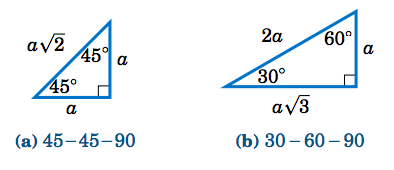Figure 1.2.2 Two general right triangles (any $$a > 0$$)

The angles $$30^\circ$$, $$45^\circ$$, and $$60^\circ$$ arise often in applications. We can use the Pythagorean Theorem to generalize the right triangles in Examples 1.6 and 1.7 and see what any $$45-45-90$$ and $$30-60-90$$ right triangles look like, as in Figure 1.2.2 above.

Example 1.10

Find the sine, cosine, and tangent of $$75^\circ$$.Solution:

Since $$75^\circ = 45^\circ + 30^\circ$$, place a $$30-60-90$$ right triangle $$\triangle\,ADB$$ with legs of length $$\sqrt{3}$$ and $$1$$ on top of the hypotenuse of a $$45-45-90$$ right triangle $$\triangle\,ABC$$ whose hypotenuse has length $$\sqrt{3}$$, as in the figure on the right. From Figure 1.2.2(a) we know that the length of each leg of $$\triangle\,ABC$$ is the length of the hypotenuse divided by $$\sqrt{2}$$. So $$AC = BC = \frac{\sqrt{3}}{\sqrt{2}} =\sqrt{\frac{3}{2}}$$. Draw $$\overline{DE}$$ perpendicular to $$\overline{AC}$$, so that $$\triangle\,ADE$$ is a right triangle. Since $$\angle\,BAC = 45^\circ$$ and $$\angle\,DAB = 30^\circ$$, we see that $$\angle\,DAE = 75^\circ$$ since it is the sum of those two angles. Thus, we need to find the sine, cosine, and tangent of $$\angle\,DAE$$.

Notice that $$\angle\,ADE = 15^\circ$$, since it is the complement of $$\angle\,DAE$$. And $$\angle\,ADB = 60^\circ$$, since it is the complement of $$\angle\,DAB$$. Draw $$\overline{BF}$$ perpendicular to $$\overline{DE}$$, so that $$\triangle\,DFB$$ is a right triangle. Then $$\angle\,BDF = 45^\circ$$, since it is the difference of $$\angle\,ADB = 60^\circ$$ and $$\angle\,ADE = 15^\circ$$. Also, $$\angle\,DBF = 45^\circ$$ since it is the complement of $$\angle\,BDF$$. The hypotenuse $$\overline{BD}$$ of $$\triangle\,DFB$$ has length $$1$$ and $$\triangle\,DFB$$ is a $$45-45-90$$ right triangle, so we know that $$DF = FB = \frac{1}{\sqrt{2}}$$.

Now, we know that $$\overline{DE} \perp \overline{AC}$$ and $$\overline{BC} \perp \overline{AC}$$, so $$\overline{FE}$$ and $$\overline{BC}$$ are parallel. Likewise, $$\overline{FB}$$ and $$\overline{EC}$$ are both perpendicular to $$\overline{DE}$$ and hence $$\overline{FB}$$ is parallel to $$\overline{EC}$$. Thus, $$FBCE$$ is a rectangle, since $$\angle\,BCE$$ is a right angle. So $$EC = FB = \frac{1}{\sqrt{2}}$$ and $$FE = BC = \sqrt{\frac{3}{2}}$$. Hence,

$DE ~=~ DF ~+~ FE ~=~ \tfrac{1}{\sqrt{2}} ~+~ \sqrt{\tfrac{3}{2}} ~=~ \tfrac{\sqrt{3} ~+~ 1}{\sqrt{2}} \quad\text{and}\quad AE ~=~ AC ~-~ EC ~=~ \sqrt{\tfrac{3}{2}} ~-~ \tfrac{1}{\sqrt{2}} ~=~ \tfrac{\sqrt{3} ~-~ 1}{\sqrt{2}} ~. ~\text{ Thus,}$

$\sin\;75^\circ = \tfrac{DE}{AD} = \tfrac{\frac{\sqrt{3} + 1}{\sqrt{2}}}{2} = \tfrac{\sqrt{6} + \sqrt{2}}{4} ~,~ \cos\;75^\circ = \tfrac{AE}{AD} = \tfrac{\frac{\sqrt{3} - 1}{\sqrt{2}}}{2} = \tfrac{\sqrt{6} - \sqrt{2}}{4} ~,~\text{and} ~ \tan\;75^\circ = \tfrac{DE}{AE} = \tfrac{\frac{\sqrt{3} + 1}{\sqrt{2}}}{\frac{\sqrt{3} - 1}{\sqrt{2}}} = \tfrac{\sqrt{6} + \sqrt{2}}{\sqrt{6} - \sqrt{2}} ~.$

Note: Taking reciprocals, we get $$\csc\;75^\circ = \frac{4}{\sqrt{6} + \sqrt{2}}$$, $$\sec\;75^\circ = \frac{4}{\sqrt{6} - \sqrt{2}}$$, and $$\cot\;75^\circ = \frac{\sqrt{6} - \sqrt{2}}{\sqrt{6} + \sqrt{2}}$$.